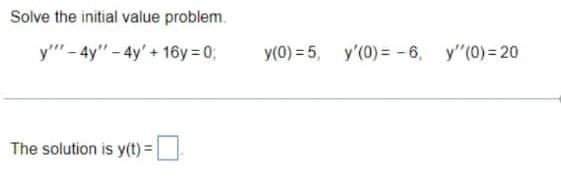Question:

# Solve the initial value problem. y"'-4y"-4y' +16y=0; y(0)

Last updated: 8/6/2022Solve the initial value problem. y"'-4y"-4y' +16y=0; y(0) = 5, y'(0)=-6, y''(0)=20 The solution is y(t) = ____